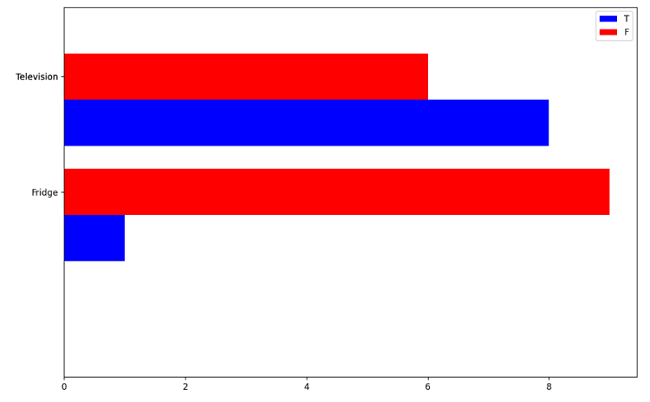# How to plot multiple horizontal bars in one chart with matplotlib?

Learn, how can we plot multiple horizontal bars in one chart with matplotlib? By Pranit Sharma Last updated : September 23, 2023

One of the important processes of data analysis is data visualization. Data visualization is a process of representing the statistical or categorical data in the form of charts, graphs, or any pictorial format.

Data visualization is an important process as far as data analysis is concerned because it allows us to understand the various kinds of patterns from the data so that we can draw some useful insights from it.

Some of the most important libraries used for data visualization are matplotlib, seaborn, plotly, bokeh, folium, plotnine, etc.

## Plotting multiple horizontal bars in one chart with matplotlib

To plot multiple horizontal bars in one chart with matplotlib, we will first import pyplot from matplotlib library and pandas library, below is the syntax:

```import pandas as pd
import matplotlib.pyplot as plt
```

Once the libraries are imported, we will then set the figure size followed by the creation of an array, followed by setting the dimensions and creating the bars, and finally followed by plotting the bars.

Let us understand with the help of an example,

## Python program to plot multiple horizontal bars in one chart with matplotlib

```# Importing pandas package
import pandas as pd

# Importing matplotlib library
import matplotlib.pyplot as plt

# Import numpy package
import numpy as np

# Setting up the size
plt.rcParams["figure.figsize"] = [10.30, 6.50]
plt.rcParams["figure.autolayout"] = True

# Create an array
array = np.array([2, 2, 1])

# Set width
width = 0.4

figure, axis = plt.subplots()

# Plot the horizontal bar
axis.barh(array, np.array([6, 8, 1]), width, color='Blue', label='T')
axis.barh(array + width, np.array([6, 6, 9]), width, color='Red', label='F')

# Setting Y-axis
axis.set(yticks= array + width, yticklabels=np.array(['Mobile', 'Television','Fridge']),
ylim=[2*width - 1, len(array)])

# Show legend
axis.legend(loc='upper right')

# Display the plot
print(plt.show())
```

### Output

The output of the above program is: